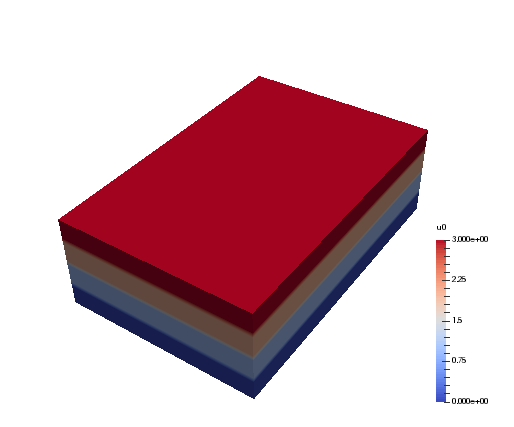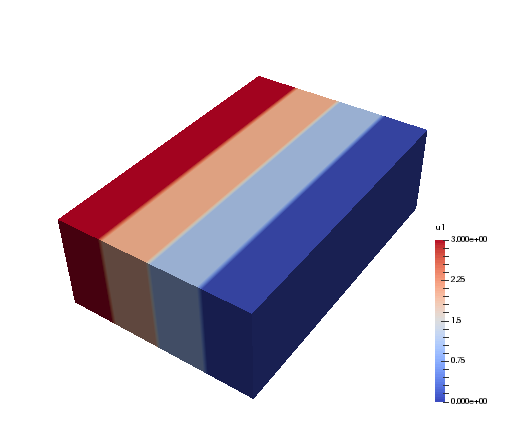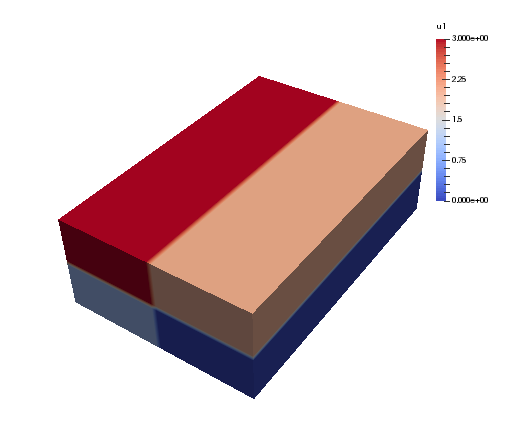# MPI¶

Shenfun makes use of the Message Passing Interface (MPI) to solve problems on distributed memory architectures. OpenMP is also possible to enable for FFTs.

Dataarrays in Shenfun are distributed using a new and completely generic method, that allows for any index of a multidimensional array to be distributed. To illustrate, lets consider a TensorProductSpace of three dimensions, such that the arrays living in this space will be 3-dimensional. We create two spaces that are identical, except from the MPI decomposition, and we use 4 CPUs (mpirun -np 4 python mpitest.py, if we store the code in this section as mpitest.py):

from shenfun import *
from mpi4py import MPI
from mpi4py_fft import generate_xdmf
comm = MPI.COMM_WORLD
N = (20, 40, 60)
K0 = Basis(N, 'F', dtype='D', domain=(0, 1))
K1 = Basis(N, 'F', dtype='D', domain=(0, 2))
K2 = Basis(N, 'F', dtype='d', domain=(0, 3))
T0 = TensorProductSpace(comm, (K0, K1, K2), axes=(0, 1, 2), slab=True)
T1 = TensorProductSpace(comm, (K0, K1, K2), axes=(1, 0, 2), slab=True)


Here the keyword slab determines that only one index set of the 3-dimensional arrays living in T0 or T1 should be distributed. The defaul is to use two, which corresponds to a so-called pencil decomposition. The axes-keyword determines the order of which transforms are conducted, starting from last to first in the given tuple. Note that T0 now will give arrays in real physical space that are distributed in the first index, whereas T1 will give arrays that are distributed in the second. This is because 0 and 1 are the first items in the tuples given to axes.

We can now create some Arrays on these spaces:

u0 = Array(T0, val=comm.Get_rank())
u1 = Array(T1, val=comm.Get_rank())


such that u0 and u1 have values corresponding to their communicating processors rank in the COMM_WORLD group (the group of all CPUs).

Note that both the TensorProductSpaces have functions with expansion

(1)$u(x, y, z) = \sum_{n=-N/2}^{N/2-1}\sum_{m=-N/2}^{N/2-1}\sum_{l=-N/2}^{N/2-1} \hat{u}_{l,m,n} e^{\imath (lx + my + nz)}.$

where $$u(x, y, z)$$ is the continuous solution in real physical space, and $$\hat{u}$$ are the spectral expansion coefficients. If we evaluate expansion (1) on the real physical mesh, then we get

(2)$u(x_i, y_j, z_k) = \sum_{n=-N/2}^{N/2-1}\sum_{m=-N/2}^{N/2-1}\sum_{l=-N/2}^{N/2-1} \hat{u}_{l,m,n} e^{\imath (lx_i + my_j + nz_k)}.$

The function $$u(x_i, y_j, z_k)$$ corresponds to the arrays u0, u1, whereas we have not yet computed the array $$\hat{u}$$. We could get $$\hat{u}$$ as:

u0_hat = Function(T0)
u0_hat = T0.forward(u0, u0_hat)


Now, u0 and u1 have been created on the same mesh, which is a structured mesh of shape $$(20, 40, 60)$$. However, since they have different MPI decomposition, the values used to fill them on creation will differ. We can visualize the arrays in Paraview using some postprocessing tools, to be further described in Sec Post processing:

u0.write('myfile.h5', 'u0', 0, domain=T0.mesh())
u1.write('myfile.h5', 'u1', 0, domain=T1.mesh())
if comm.Get_rank() == 0:
generate_xdmf('myfile.h5')


And when the generated myfile.xdmf is opened in Paraview, we can see the different distributions. The function u0 is shown first, and we see that it has different values along the short first dimension. The second figure is evidently distributed along the second dimension. Both arrays are non-distributed in the third and final dimension, which is fortunate, because this axis will be the first to be transformed in, e.g., u0_hat = T0.forward(u0, u0_hat).We can now decide to distribute not just one, but the first two axes using a pencil decomposition instead. This is achieved simply by dropping the slab keyword:

T2 = TensorProductSpace(comm, (K0, K1, K2), axes=(0, 1, 2))
u2 = Array(T2, val=comm.Get_rank())
u2.write('pencilfile.h5', 'u2', 0)
if comm.Get_rank() == 0:
generate_xdmf('pencilfile.h5')


Running again with 4 CPUs the array u2 will look like:The local slices into the global array may be obtained through:

>>> print(comm.Get_rank(), T2.local_slice(False))
0 [slice(0, 10, None), slice(0, 20, None), slice(0, 60, None)]
1 [slice(0, 10, None), slice(20, 40, None), slice(0, 60, None)]
2 [slice(10, 20, None), slice(0, 20, None), slice(0, 60, None)]
3 [slice(10, 20, None), slice(20, 40, None), slice(0, 60, None)]


In spectral space the distribution will be different. This is because the discrete Fourier transforms are performed one axis at the time, and for this to happen the dataarrays need to be realigned to get entire axis available for each processor. Naturally, for the array in the pencil example (see image), we can only perform an FFT over the third and longest axis, because only this axis is locally available to all processors. To do the other directions, the dataarray must be realigned and this is done internally by the TensorProductSpace class. The shape of the datastructure in spectral space, that is the shape of $$\hat{u}$$, can be obtained as:

>>> print(comm.Get_rank(), T2.local_slice(True))
0 [slice(0, 20, None), slice(0, 20, None), slice(0, 16, None)]
1 [slice(0, 20, None), slice(0, 20, None), slice(16, 31, None)]
2 [slice(0, 20, None), slice(20, 40, None), slice(0, 16, None)]
3 [slice(0, 20, None), slice(20, 40, None), slice(16, 31, None)]


Evidently, the spectral space is distributed in the last two axes, whereas the first axis is locally avalable to all processors. Tha dataarray is said to be aligned in the first dimension.